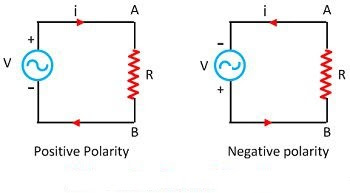## ELECTRICAL BASICS

### Electric Engineering

It is the branch of engineering that deals with the technology of electricity, applications of equipment,devices and system which use electricity, electronics and electromagnetism.

Electric voltage
• Electrical potential energy per unit charge.
• Potential difference between two points in electrical circuit.
• Voltage is a pressure which pushes the charged electrons means current.
• Measured in joules/coulomb
• SI unit volt (V)
• V = I . R

Electric Current
• Rate at which charge is flow from one terminal (positive) to the another terminal (negative).
• Measured in ampere (A)
• SI unit ampere
•  I = V/R
Alternating Current (AC)
• The current that changes its magnitude and polarity at regular intervals of time is called an alternating current.
• The major advantage of using the alternating current instead of direct current is that the alternating current is easily transformed from higher voltage level to lower voltage level.
• When the resistive load R is connected across the alternating source shown in the figure below, the current flows through it. The alternating current flows in one direction and then in the opposite direction when the polarity is reversed.Alternating Current Circuit

• The alternating current produces low iron and copper losses in AC rotating machine and transformer because it improves the efficiency of AC machines.
• The alternating current offer less interference to the nearby communication system (telephone lines etc.).
• They produce the least disturbance in the electrical circuits.

Direct Current (DC)
• Direct Current, which is electrical current that flows in one direction.
• In dc circuits, the current is in one direction unlike the alternating current (AC) where the current reverses direction 50 or 60 times a second depending on the frequency of the supply.
Sources of Direct Current
• DC generators
• Batteries
• DC power converters that rectify the AC
• Solar panels
• Thermocouples
• Most equipment are more efficient when directly powered from DC.
• More efficient especially when the power leads are less than 100 feet.
• Less risks of shock hazard for voltages below 48 Volts.
Applications of Dc

DC is used in almost all electronics equipment, electric vehicles, automation, control of electrical equipment, and more.
Most office and domestic equipment such as TVs, audio systems, amplifiers, flash lights, computers, tablets and smartphones use DC power to function. However, since the universally available power is AC, the equipment uses an external or internal power supply to convert the utility mains AC into the desired DC for the equipment.

Resister

• Materials tendency to resist the flow of charge.
• The effect of resistor is known as resistance.
• Measured in ohm (R)
• SI  unit ohm
• R = V/I
Capacitor
• Capacitor stores energy in electrical field.
• Passive electronic component with two terminals.
• The effect of capacitor is known as capacitance.
• Its SI unit is Farads
• Denoted as E.
Inductor

• An inductor, also called a coil, choke, or reactor.
• is a passive two-terminal electrical component that stores energy in a magnetic field when electric current flows through it.
• An inductor typically consists of an insulated wire wound into a coil around a core.
• Its SI unit is Henries (H).
Electric Power
• Electric power is the rate, per unit time, at which electrical energy is transferred by an electric circuit.
• The SI unit of power is the watt, one joule per second.
Power Efficiency
• Ratio of the output power into the input power is called power efficiency.
• Measured in watt (P).
• P=E/t
Power Factor
• The power factor is equal to the real or true power P in watts (W) divided by the apparent power |S| in volt-ampere (VA)
Ohm's Law
• Ohm's law states that the current through a conductor between two points is directly proportional to the voltage across the two points  inversely proportional to the resistance, at the constant temperature.
• I= V/R
Kirchoff's current law
• Total current entering into the junction is equal to the total current leaving the junction.
Kirchoff's voltage law
• Algebraic sum of the voltage drop in closed loop is zero.

unknown said...

Wow, cool post. I’d like to write like this too – taking time and real hard work to make a great article… but I put things off too much and never seem to get started. Thanks though. thermal imaging company

servicecorrp said...

I think this is an informative post and it is very useful and knowledgeable. therefore, I would like to thank you for the efforts you have made in writing this article. Test and Tag cost Adelaide

Dexter Magnetic Technologies said...

Enjoy reading the article above , it really explains everything in detail,the article is very interesting and effective.Thank you and good luck for the upcoming articles. Magnetic core material